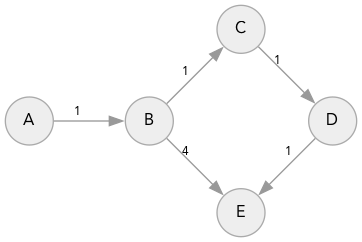# Reprioritize operation in Dijkstra’s Algorithm

from Red Blob Games

{ this post published here ; I am not sure whether this also applies if you use an inadmissible heuristic }

I’m working on a tutorial showing how Breadth First Search, Dijkstra’s Algorithm, Greedy Best-First Search, and A* are related. I’m focusing on keeping the code simple and easy to understand.

While tracking down a bug in my Dijkstra’s Algorithm code, I realized I had forgotten to implement the “reprioritize” case. That led me to wonder why my code seemed to work without handling that case. It turns out that case is never triggered by the graphs I’m searching over. Let me explore this …

Algorithm textbooks describe the algorithm as starting with $$\infty$$ everywhere and then checking the new cost against the old cost. The old cost is always defined so it’s a single test:

if cost_so_far[current] + cost(current, next) < cost_so_far[next]:
cost_so_far[next] = cost_so_far[current] + cost(current, next)
came_from[next] = current
frontier.reprioritize(next, cost_so_far[next])


In practice I don’t initialize the priority queue to have all vertices with distance set to $$\infty$$. Instead, I start the priority queue with only the start element, and only insert elements when their distance is finite. Keeping the priority queue small makes it significantly faster, especially with early exit.

However, this complicates the code. I now have two cases instead of one:

1. The new cost is less than the old cost because the old cost was $$\infty$$, and we need to insert the node into the priority queue. This is a simpler operation, and it’s more common, so it’s worth separating out.
2. The node wasn’t already in the priority queue, and has no old cost, and we need to reprioritize the node already in the priority queue. This is rare and more complicated than an insert.

This logic can go either in the algorithm code or in the priority queue code. I had put it in the algorithm (but in the tutorial I am going to instead put it in the priority queue, because it keeps the algorithm easier to understand).

if next not in cost_so_far:
cost_so_far[next] = cost_so_far[current] + cost(current, next)
came_from[next] = current
frontier.insert(next, cost_so_far[next])
elif cost_so_far[current] + cost(current, next) < cost_so_far[next]:
cost_so_far[next] = cost_so_far[current] + cost(current, next)
came_from[next] = current
frontier.reprioritize(next, cost_so_far[next])


I wanted to better understand which situations lead to the reprioritize case. Let’s say we’re looking at edge $$b \rightarrow c$$ and we previously found $$c$$ via edge $$a \rightarrow c$$. (Note: algorithm textbooks call this $$g$$ but I call it cost_so_far)

1. To trigger the reprioritize case, I need the old cost to be worse than the new cost: $$g(a) + \mathit{cost}(a,c) > g(b) + \mathit{cost}(b,c)$$.
2. Dijkstra’s Algorithm explores nodes in increasing order. Since $$a$$ was explored before $$b$$, $$g(a) \leq g(b)$$, or equivalently, $$g(b) - g(a) \geq 0$$

Those two together can be rewritten as $$\mathit{cost}(a,c) - \mathit{cost}(b,c) > g(b) - g(a) \geq 0$$

That means when $$\mathit{cost}(a,c) - \mathit{cost}(b,c) > 0$$ we need to reprioritize.

In several of my game projects, $$\mathit{cost}(x,y)$$ depends only on $$y$$. That means $$\mathit{cost}(a,c) = \mathit{cost}(b,c)$$, and we never need to reprioritize.

That means any bugs in my reprioritize code never mattered!

It also means the abstraction boundaries between the graph data structure, the search algorithm, and the priority queue data structure potentially make the code more complicated and hurting optimization opportunities. I think this rule works, but I’m not sure: if we have a monotonic ordering (always with Dijkstra’s algorithm, and A* with a consistent heuristic), and the movement cost only depends on the target node, then we never need to reprioritize. And that means we can use a simpler and faster regular priority queue instead of a reprioritizable priority queue.

## 1  Testing#

Let’s define a graph where we actually need to reprioritize, then try it out.class Graph:
def __init__(self):
self.edges = {}
self.weights = {}

def neighbors(self, id):
return self.edges.get(id, [])

def cost(self, a, b):
return self.weights.get((a, b), 1)

self.edges.setdefault(a, []).append(b)
self.weights[(a, b)] = w

class PriorityQueue:
def __init__(self):
self.elements = {}

def empty(self):
return len(self.elements) == 0

def put(self, item, priority):
if item in self.elements:
print("Reprioritizing", item, "from", self.elements[item], "to", priority)
else:
print("Inserting", item, "with priority", priority)
self.elements[item] = priority

def get(self):
best_item, best_priority = None, None
for item, priority in self.elements.items():
if best_priority is None or priority < best_priority:
best_item, best_priority = item, priority

del self.elements[best_item]
return best_item

def search(graph, start):
frontier = PriorityQueue()
frontier.put(start, 0)
came_from = {}
cost_so_far = {}
came_from[start] = None
cost_so_far[start] = 0

while not frontier.empty():
current = frontier.get()

for next in graph.neighbors(current):
new_cost = cost_so_far[current] + graph.cost(current, next)
if next not in cost_so_far or new_cost < cost_so_far[next]:
cost_so_far[next] = new_cost
frontier.put(next, new_cost)
came_from[next] = current

g = Graph()

Inserting A with priority 0